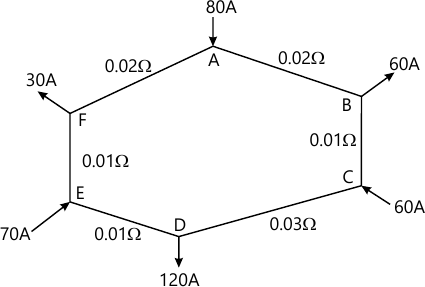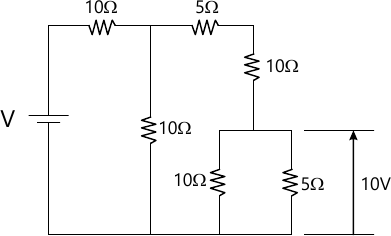MORE IN Basic Electrical Engg.
VTU First Year Engineering (P Cycle) (Semester 2)
Basic Electrical Engg.
December 2014
Total marks: --
Total time: --
INSTRUCTIONS
(1) Assume appropriate data and state your reasons
(2) Marks are given to the right of every question
(3) Draw neat diagrams wherever necessary

1(a) A 20V battery with an internal resistance of 5?is connected to a resistor of x ohms. If an additional 6?resistor is connected across the battery, find the value of x so that the external power supplied by the battery remains the same.
6 M
1(b) Find the current in all the branches of the network shown.6 M
1(c) Two coils, X of 12000 turns and Y f 15000 turns, lie in parallel planes so that 45% of the flux produced by coil X links coil Y. A current of 5 A in X produces 0.05Wb while the same current in Y produce s 0.075Wb. Calculate (a) the mutual inductance, (b) the coupling coefficient, and (c) the percentage of flux produced by coil Y and linking with coil X.
8 M

2(a) Calculate the supply voltage V in the circuit shown.6 M
2(b) A coil of resistance 150?is placed in a magnetic field of 0.1mWb. The oil has 500turns and a galvanometer of 450?is connected in series with it. The coil is moved in 0.1s from the given field to another field of 0.3mWb. Find the average induced emf and the average current through the coil.
6 M
2(c) In the circuit shown what is the voltage across AB if
i. Switch is open
ii. Switch is closed8 M

3(a) Explain the principle of operation of dc motor.
6 M
3(b) Explain with a neat diagram, the constructional fea tures and operation of an induction type single phase energy meter.
6 M
3(c) A shunt generator delivers 50 kW at 250 V and runs at 400 rpm. The armature and field resistances are 0.02? and 50? respectively. Calculate the speed of the machine running as a shunt motor and taking 50 kW input at 250 V. Allow 1 V per brush for contact drop.
8 M

4(a) Deduce the relation between induced emf and terminal voltage of separately excited, shunt and series generators.
6 M
4(b) Explain with a neat diagram, the constructional fea tures and operation of dynamometer type wattmeter.
6 M
4(c) A lap wound 750 rpm shunt motor has an armature resistance of 0.4?and shunt field resistance of 200? respectively. The armature has 120 coils each of 3 turn each. The flux per pole is 0.03Wb. I f the load resistance is 10? determine the terminal voltage.
8 M

5(a) Obtain an expression for the voltage across p ure inductor, if the current through it is $i=I_{m}sin\omega t\$
6 M
5(b) Write a short note on (i)Fuse and (ii) Electric sho ck.
6 M
5(c) A coil of resistance 10?and inductance 0.1 H is connected in series with a 150 ?F capacitor across a 200 V, 50 Hz supply. Calculate the voltage across the coil and the capacitor respectively.
8 M

6(a) Deduce a condition at which an RLC circuit behaves like a resistive circuit. State whether the current in the circuit is minimum or maximum.
6 M
6(b) Find the expression for the current, and calculate the power, when a voltage represented by v=283 sin 100?t is applied to a coil of 50? resistance and 0.159 H inductance.
6 M
6(c) Write a short note on (i)Miniature Circuit Breaker and (ii) Earth leakage circuit breaker.
8 M

7(a) Explain the advantages of rotating field type a lternator.
6 M
7(b) For a certain load, one wattmeter read 20 kW and the other 5 kW after the voltage circuit of the wattmeter has been reversed. Calculate the phaseangle between voltage and current.
6 M
7(c) A 12 pole, 500rpm star connected alternator has 60 slots with 20 conductors/slot. The flux per pole is 0.02Wb and sinusoidally distributed. The winding factor is 0.97. Calculate frequency, phase emf and line emf.
8 M

8(a) Show that two wattmeters are sufficient to measure three phase power.
6 M
8(b) A 3 phase, 6 pole star connected alternator revolve s at 1000 rpm. The stator has 90 slots and 8 conductors per slot. The flux per pole is 0.05Wb. Calculate voltage generated, if $k_{w}=0.96\$
6 M
8(c) Three similar coils each having resistance of 10? and reactance 8?are connected in star across a 400V, 3 phase supply. Determine the line current, total power and reading of each wattmeters connected to measure power.
8 M

9(a) What are the losses in a transformer and how they v ary with load? Deduce a condition for maximum efficiency.
6 M
9(b) A 6 pole alternator runs at 1000 rpm, and supplies power to a 4 pole, 3 phase induction motor. The frequency of rotor of induction motor is 2 Hz. Determine the slip and speed of the motor.
6 M
9(c) A 40kVA single phase transformer has core loss of 4 50W and full load copper loss of 850W. If the power factor of the load is 0.8, calculate (i) full load efficiency, (ii) load corresponding to maximum efficiency, and (iii) maximum efficiency at unity power actor.
8 M

10(a) Explain the working principle of a 3 phase induction motor.
6 M
10(b) Obtain an expression for the load KVA at which maxi mum efficiency occurs.
6 M
10(c) Explain why it is not advisable to start a 3 phase induction motor by directly connecting it across the supply. With a circuit diagram, explain a star-delta starter.
8 M

More question papers from Basic Electrical Engg.